Reach Us+32-10-28-02-25
The Double Slit Experiment-Explained | OMICS International
Journal of Physical Mathematics
All submissions of the EM system will be redirected to Online Manuscript Submission System. Authors are requested to submit articles directly to Online Manuscript Submission System of respective journal.

# The Double Slit Experiment-Explained

Yousif ME*

Department of Physics, The University of Nairobi, Kenya

*Corresponding Author:
Yousif ME
Professor, Department of Physics
The University of Nairobi, Kenya
Tel: +254 20 318262
E-mail: [email protected]

Received Date: March 07, 2016; Accepted Date: May 24, 2016; Published Date: June 01, 2016

Citation: Yousif ME (2016) The Double Slit Experiment-Explained. J Phys Math 7:179. doi:10.4172/2090-0902.1000179

Copyright: © 2016 Yousif ME. This is an open-access article distributed under the terms of the Creative Commons Attribution License, which permits unrestricted use, distribution, and reproduction in any medium, provided the original author and source are credited.

Visit for more related articles at Journal of Physical Mathematics

#### Abstract

The wavelet envisioned by Huygen’s in diffraction phenomenon is re-interpreted as being polarized after passing through slit/hole which removed the electric field component from the Electromagnetic Radiation (EM-R), the remained wave consist of the Circular Magnetic Field (CMF), this CMF lost the speed of light and the electric field, hence it’s a short distance travel field, originated from the CMF produced by accelerated electrons, integrated with the Electric Field (EF) during the Flip-Flop (F-F) mechanism producing EM-R; hence the passing of light through a single hole/slit resulted in a CMF which reproduced as rings on the monitor screen in single wave diffraction, while the interference of two such CMF in double slits experiment, produced constructive or destructive interference forming patches on the monitor screen; and the perceived electron diffraction is an enter of two CMF from a single electron into a slit then emerged to produce constructive or destructive interference, in addition to the electron which entered and emerged from the slit with the stronger CMF, the paper finally derived the origin of Planck ‘constant (h) for the second time; the logical interpretation of double slits diffraction will restore the common sense in the physical world, distorted by the pilot wave.

#### Keywords

Double slit experiment; wavelet; Circular magnetic field; Electron diffraction; Polarization; Origin of Planck’ constant

#### Introduction

In his explanation to the photo electric effect in 1905, Einstein invoked quanta (photon) as theoretical justification to expel electron from the atom , which was viewed as a particle with zero rest mass , although the idea was rejected by many of his contemporary scientists lead by Millikan, , also famous were J.J. Thomson, Summerfield, and Richardson , but with endorsement from Compton experiment in 1922 , scientists gradually accepted the notion that electromagnetic radiation is wave particle duality .

Contrary to light, where the discovery of diffraction preceded the wave theory, the electron diffraction was discovered as a consequence of a deliberate attempt to prove the wave nature of the electron , after de Broglie extended duality to particles in 1924 , then Davisson and Germer, explained the diffraction peak wave, generated by “electron wave,” as the wavelength of Bragg formula, and resulted in diffraction pattern [9,10], that was confirmed differently by G. P. Thomson ; although the reflected electrons from the nickel crystal in Davisson and Germer experiment, occurred before detection of diffracted beams , which posed contradiction on how deflected electron could store reflected phantom wave? Regardless of that, both experiments became decisive in endorsing wave particle duality, making it acceptable, leading to new form of physics, contradicting the common sense and norm of life . The ambiguity and uncertainty in this “electron wave,” or “phantom wave” brought great confusions; which led some to introduced the pilot wave, thought as similar to electromagnetic field , a state of confusion led Einstein to express at several occasions, before his death in 1955, that “for fifty years, he failed to understand what quanta (photon) is”  which amount to doubt on photon’s existence, but the wave particle duality became acceptable by lack of sound alternative. The re-interpretation of Photoelectric Effects, suggested the Magnetic Radiation Force (FmR) as embedded in Electromagnetic Radiation (EM-R) , similar in nature to Planck’ Radiation Energy (EmR) , while the production of Secondary Electromagnetic Radiation (S-EM-R) in “The Compton Effect Re- Visited” , consolidated the existence of an alternative interpretation base. This is based on exploring the characteristics of the Circular Magnetic Field (CMF) produced by energetic electrons , and the Spinning Magnetic Force (SMFc) produced by Spinning Magnetic Field (SMF), , it helped elaborating many phenomena, and subjected the double slit experiment into new analysis.

This paper is the fifth among series intended to prove the correctness of our “The Magnetic Interaction”  during a discussion , and since light represents more subtle and elusive problems than most other aspects of physical experience , and J. J. Thomson realized that the detection of a train of waves associated with the movement of electrons was not predicted by Maxwell’s equations, emphasizing that, such a view of the electron had to be wrong , thus the existence of CMF produced by electron, not predicted by Maxwell’s equation cast doubt about the acceleration mechanism for EM-R generation, and gives weight to the Flip-Flop (F-F) mechanism describing the transverse light wave mechanism , it helped explored and elaborated radiation energy, conditions initiating EM-R generation and the nature and characteristics of Planck’ Constant (h) , it explained the Radiation Magnetic Force (FmR) embedded in EM-R, a phenomenon puzzled Einstein for fifty years , while the Planck’ was explained as a parameter of constant elements within the energy transformation process , and the Compton Effect was interpreted as a production of Secondary Electromagnetic Radiation (S-EM-R) phenomenon , all these prepared the ground for the existence of an alternative interpretation in micro-physical world, based on wave matter interactions.

Therefore, the diffraction of ripples through a single narrow opening, and two narrow openings , which was the bases for Huygen’s principle of diffraction, explained with semi-circle water waves generated in pond , is reinterpreted as a Circular Magnetic Field (CMF) formed from this wave, and resulted from the polarized Electromagnetic Radiation (EM-R) when passed through a small hole/ slit, thus removing the electric field quantity, leaving the magnetic field, hence the diffraction is interpreted as the interference of the resulted CMF which neither travel with speed of light, nor carrying electric field, the CMF is not semi-circle, rather it is a full circle, and composed of the magnetic portion of EM-R, it is the Circular Magnetic Field (CMF), which originated in the Flip-Flop mechanism and EM-R production .

Since Electron diffraction is the strongest evidence for the principles of wave-mechanics on which the whole of atomic physics is based , hence relating the CMF and EM-R characteristics to Young's double slit experiment, and the reinterpretation of wavelets as CMF, formed in single slit diffraction in CMF shape, and the interference of two such CMF in double slits experiment, resulted in constrictive or destructive interference; while electron diffraction is interpreted as the interference of two CMF entered and emerged from two slits and originated from a single electron, which accompanied the strongest CMF through one of the two slits, the paper finally derived the origin and structures of Planck’ constant (h) for the second time .

As the understanding of the dynamical processes in chemistry, materials science and biology on micro scale stems almost exclusively from time-resolved spectroscopy  which emerged from the diffraction carried by Bragg and based on Huygen’s idea , therefore by elaborating the true mechanism of this field, would enrich and expand human understanding and unified the general level of human vision. In tackling this, it is better remember that, till early fifteen centaury, the Geocentric Model of the universe  was believed to reflect the true reality of the celestial bodies, forming an enforced doctrine, no lesson learned; and for nearly a century the duality dogma rejected all attempts to correct its oddness which diverted general scientific mentality from the common sense pattern, thus limiting the scope of human endeavor.

#### Energetic Circular Magnetic Field (CMF)

The Circular Magnetic Field (CMF) produced by electrons in conductor carrying electric current originated from the magnetic phenomenon discovered by Hans Christian Oersted in 1819 , after which André Ampère determined the circular shape of the magnetic field around the conductor, and derived related formula using electric parameters for a force between two conductors carrying electric current , and emphasis was directed towards electric field , thus the outweigh of electric parameters demised the CMF, where Maxwell equations in unified electric and magnetic fields, claimed both quantities equally contribute to the total energy density of the Electromagnetic Wave (EM-Wave) , but how this could be true when electric field in electron is not variable, while the magnetic field component designated as Circular Magnetic Field (CMF) increased with velocity or energy [35-37], and given as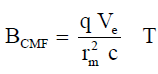(1)

Where, rm is the magnetic radius in meter, c is the velocity of light in m.s-1, Ve is the electron velocity in m.s-1 and BCMF is the CMF (B2e) in T.

The energy of Electromagnetic Radiation (EM-R) is shown to concentrate in the CMF (BCMF ) , this is why the magnitude of CMF (BCMF) energy in Electromagnetic Radiation (EM-R) increased rapidly with frequency, because frequency is part of CMF formation  given as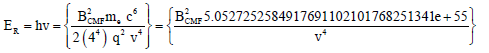(2)

Where, v is the Electromagnetic Radiation Frequency in Hz, me is electron mass in kg, q is charge in Coulomb, h is Planck’ constant in J.s., and the Radiation Energy ER is in Joules. Table 1 give the variation of radiated energy with the CMF (BCMF); using Eq(2) or Eq(21), this variation is also showed in the Secondary Electromagnetic Radiation (S-EM-R) , in which energetic CMF (BCMF) interacted with strong Nucleus Spinning Magnetic Field (B1U)  producing EM-R , both magnitudes of the CMF and the Nucleus Spinning Magnetic Field (B1U) are given in Table 1, the B1U is derived by 

ve(m.s-1) ER(J) v(Hz) tF(s) γ(m) rm(λ/4)(m) BCMF(T) B1U(T)
1.2061422956252779358797823011235e+5 6.6260755e-21 1.0e+13 1.e-13 3.e-5 7.5e-6 1.1451578327733205830019441826343e-12 7.1447751068120606978208204881839e+2
3.8141568364400215819565442948061e+6 6.6260755e-18 1.0e+16 1.e-16 3.e-8 7.5e-9 3.6213070319459084345608961862395e-5 7.1447751068120606978208204881839e+5
1.2061422956252779358797823011235e+7 6.6260755e-17 1.0e+17 1.e-17 3.e-9 7.5e-10 1.1451578327733205830019441826343e-2 7.1447751068120606978208204881839e+6
1.2061422956252779358797823011235e+8 6.6260755e-15 1.0e+19 1.e-19 3.e-11 7.5e-12 1.1451578327733205830019441826343e+3 7.1447751068120606978208204881839e+8
6.606313428750293606328455488465e+9 1.98782265e-11 3.e+22 3.3333333333333333333333333333333e-23 1.e-14 2.5e-15 5.6450589922230883450151444893508e+11 2.1434325320436182093462461464552e+12
ve(m.s-1) ER(J) f(Hz) tF(s) λ(m) rm(λ/4)(m) BCMF(T) B1U(T)

Table 1: The Circular Magnetic Field (BCMF) produced by energetic electron represents Electromagnetic Radiation Energy (ER), carried by wave of specific frequency.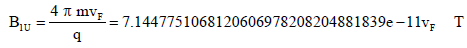(3)

Where, VF is Flipping Frequency (VF) or radiation frequency in Hertz, and B1U is the nucleus Spinning Magnetic Field (or the strong field). Substituting the equivalent of v in Eq (2) with v in Eq (3), the frequency is given by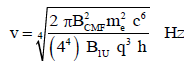(4)

Or as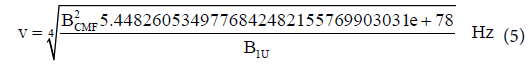Therefore, and as given by Eq (4), the radiation energy and frequency for each CMF (BCMF) given in Table 1 is related to the magnitude of Nucleus Spinning Magnetic Field (B1U) which trigger it  as given by Eq.(3), the same is the conclusion of high energy scientists, that the shortest radiation/particle bursts of x-rays and γ-rays are produced by the highest power laser , substuting v in Eq(3) with v in Eq(4) the relation between BCMF and B1U is given by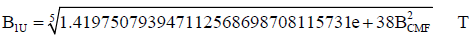(6)

The table shows from left, electron’s velocity (Ve), radiation energy (ER), frequency (v), Flipping Time (tF), wavelength (λ), the magnetic Radius (rm = λ/4), CMF (BCMF), and the Nucleus Spinning Magnetic Field (B1U), which triggers the release of the radiation .

As the CMF is resulted from moving charge as given by Eq(1), hence this equation can derive a fast moving nuclei with electric charges in Relativistic Heavy Ion Collider (RHIC) and Large Hadrons Collider (LHC) created extreem strongest magnetic fields , given in Table 2, and compared with varrities of magnetic fields; this is also to be compared with the CMF in Table 1; the CMF at frequency of 3.e+22 Hz in Table 1, contained 5.6450589922230883450151444893508e+11 Tesla, and required nucleus strong field of 2.1434325320436182093462 461464552e+12 Tesla to trigger it and pull and radiated EM-R  after the end of F-F mechanism .

Thesource(Realizationas) StrengthTesla
Earth’smagneticﬁeld 6.e-5
Atypicalhand-heldmagnet 1.e-2
SuperconductingmagnetsinLHC 8.3e+0
Surfaceﬁeldofneutronstars ~1.e+8
Criticalmagneticﬁeldofelectrons 4.e+9
Surfaceﬁeldofmagnetars ~1.e+11
Noncentralheavy-ioncoll.atRHIC ~1.e+13
Noncentralheavy-ioncoll.atLHC ~1.e+14

Table 2: Comparison of magnitudes of several sources of Magnetic fields .

Circular Magnetic Field (CMF) Wavelet

Young’s famous “double-slit experiment” provided convincing evidence that light does have properties explainable only in terms of waves , and the diffraction of ripples through a single and two narrow openings [32,22], was the base behind Huygen’s principle of diffraction, explained in connection with the semi circle water waves generated in pond , and the pattern resulted from the superposition of diffracted waves from both slits is interpreted as that produced by two point sources vibrating in phase ; but the produced circular wave train in water, and the overlapping interference by two ripple pattern produced by two vibrating points source in phase , clearly shows the circular nature of the produced waves, and the resulted interference produced a diffraction , therefore Young’s double slits experiment shown in Figure 1A, in which light is shown to propegate from the source to the two slits, where the propegated wave is alway percieved as consisting of sinsodial shape ilustrated in Figure 1B with slits in turn produced wavelets, acting as secondary waves or sources of light according to Huygens’ construction , while the practical requirements for narrow slits which are the source of just one Huygens’ wavelet are difficult if not impossible to achieve , and since diffraction is “the pattern of beams which occur when light passes through pinholes and nets or is reflected from graduated rulers,”  and the geometrical conditions for constructive/destructive interference which apply to just one wavetrain apply to all wavetrains, and the resulted patterns of light and dark on the screen is the diffraction pattern , therefore, these showed the diffraction been conceived from the geometrical perspective not the physical dynamics of the slits on the emerged waves, thus complicated the single aperture pattern; therefore, re-studying the mechanism behind the entering and emergence of light from a hole/ slit, suggested different mechanism; and since water wave composed of single wave, while Electromagnetic Wave (EM-W) consist of electric and magnetic fields , unfortunately the shape of this wave have been mixed with semi-circle water waves generated in pond, interpreted as synonymous to magnetic wave , as shown in Figure 1A; but since it was discovered that, the electric field (E-F) produced in series of timelapse photographs, is always either pointing up or down , similar to the circularly polarized light , and since both experiments showed electric field as raising and falling along the propogating path and interpreted as moving vertically, and as polarization of light is the filtering of one component of the incident beam , thus the perceived double slit shape in Figure 1A, is neither the correct shape of EM-R entering the slit, nor the correct shape emerging from the slit, therefore the true shape of EM-R entering the slit is the one shown in Figure 1C; hence the passing of EM-R through a slit/hole, removed it’s electric field due to polarization process, hence the slit as a filter component restrained the electric field, hence the wavelet entering the slit is part of the Circular Magnetic Field (CMF) designated as δ-CMF shown in Figure 2A, therefore the emerged wavelet is re-interpreted as a change in the EM-R, where the resulted wave neither travel with speed of light, nor carrying electric field, the wave is not semi-circle, rather it is a full circular wave, and composed of the magnetic part of the EM-R, this is designated as the Circular Magnetic Field (CMF), which originated from the Circular Magnetic Field (CMF) produced by accelerated electrons  as given by Eq.(1), and shown in Figure 2B, therefore the CMF (or BCMF) as magnetic part of electromagnetic radiation contained the radiation energy given by Eq (2) and shown in Table 1, hence from Eq (2), the CMF (BCMF) of this energy is given by

Figure 1: Young’ Double Slits experiment is in (A), showing the wavelet that enters the slits, and acts as secondary sources , the perceived radiation wave is shown in (B), while (C) shows the true shape of Electromagnetic Wave (EM-W) .

Figure 2: In(A) Electromagnetic Radiation (EM-R) emerged from single slit as a polarized Circular Magnetic Field (CMF), (B) shows the Flip-Flop (F-F) transformation of both CMF-Electric Field (CMF-EF) forming EM-R  then back to the polarized CMF, (C) shows single diffracted CMF from three different holes sizes , while (D) shows two polarized CMF resulted from wavelets δ-CMF-1 and δ-CMF-2 of the EM-R, the geometrical structure of line x-x is on left of the slit line, interference of both CMF produced diffracted patches.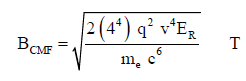(7)

Replacing ER in Eq(7) with v h, hence BCMF is given in terms of frequency as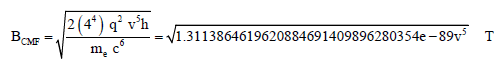(8)

The CMF given by Eq(8) is to be derived in term of frequency, the BCMF is given by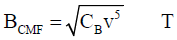(9)

Where,(CR) is the constant of radiation it is equal to 1.3113864619620884691409896280354e-89 T2. Hz-5 (T2. s5).

The CMF (BCMF given by Eq(9), is in terms of frequency, while the frequency v in Eq (8) can be written as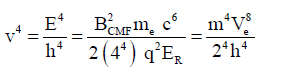(10)

From Eq (10) the following is derived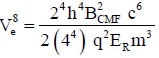(11)

Since velocity is given in term of energy as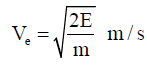(12)

For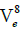the above value is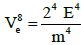(13)

Substituting the left hand side of Eq.(11) with the right hand side of Eq(13)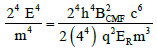(14)

Re-arranging Eq.(14), the CMF (BCMF) is given by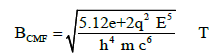(15)

From Eq(15), the CMF (BCMF) can be given by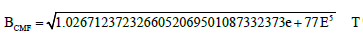(16)

Therefore, from Eq(15) the EM-R energy is given by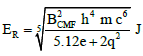(17)

Since the CMF (BCMF) is the only variable in Eq (15), therefore the EM-R energy in terms of the CMF (BCMF) is given by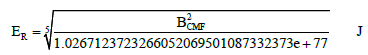(18)

Therefore, the energy of the EM-R entering the slit in Figure 2A, is contained in the CMF (BCMF) as given by Eq(18), and it’s value can be derived using Eq(16).

#### Young's Experiment and Circular Magnetic Field (CMF)

The electromagnetic wavelet entered and emerged from a small hole comparable to its wavelength is shown in Figure 2A; as explained above, this wavelet is the magnetic part of the radiation as given by Eq(15), and since a wavelet emerging from a slits in turn act as secondary wave or source of light according to Huygens’ , but as shown in the polarization process it’s a source of wave, but not light, therefore any such δ-CMF emerging from a small hole or slit, is suggested to restored to its CMF origin; therefore as shows in Figure 2B, the transformation of the Circular Magnetic Field-Electric Field (CMF-EF) into Electromagnetic Radiation (EM-R) through the Flip-Flop (F-F) mechanism , is opposite to re-transformation of the EM-R back into CMF through the polarization mechanism attained through the hole (aperture)/slit. The change of the magnetic wavelet with dimension is synonymous to the restoration of the field into the CMF, but without electric field, this is expressed by

CMF = (CMF + EF) − (EE) T (19)

Since the CMF (BCMF)is the only variable in the radiation energy given by Eq(17), and the formula shows CMF (BCMF) as the main energy in Electromagnetic Radiation (EM-R) shown in Figure 1C , and as diffraction pattern from a single slit is a central maximum with much fainter bands of half the width of the central maximum on each side, and diffraction pattern from a circular hole or aperture is, correspondingly, a central disc surrounded by much fainter rings or haloes  shown in Figure 2C, therefore these characteristics imply on the resulted CMF showed in Figure 2A, and resulted in the diffraction pattern shown in Figure 2C , which is representation of the CMF (BCMF) as it emerged from aperture of Figure 2A; while the Poisson's or Arago spot shown as a white spot in each of Figure 3C circles, interpreted to the existence of diffracted point sources at phase in the central axis, so the waves will add up and create a bright spot at the center of the image , but as shown in the figure this spot is part of the resulted CMF emerged from EM-R after the removal of the electric field, and it is even existed in the CMF-EF shown in Figure 2B, before the polarization.

The Young's double-slit experiment is basically, involves splitting a single beam of light into two beams in order to ensure that they are in phase, then allowed to overlap, and the two wave trains interfere, constructively in some places and destructively in others , the diffraction pattern is taken to be created by the interference of waves traversing two clearly separated paths , but as shown in Figure 2A, the emerged polarized wave lost its electric field, it represents the CMF shown in Figure 2B and 2C; therefore what really takes place in Young’s double slit experiment shown in Figure 2D, is that both -BCMF-1 and δ-BCMF-2 entered slit-1 and slit-2 respectively, they both transformed into BCMF-R1 and BCMF-R2 waves; therefore, the Young's double-slit experiment is an interference carried by two intense Circular Magnetic Field (CMF) as shown in Figure 2D, the magnitudes of these CMF is derived in Table 1.

The d in Figure 2D, is the distance of diffraction grating, and since line x-x is parallel to the slit line, therefore the geometrical conditions are constant for constructive/destructive interference which apply to both wave train and apply to all wave trains, and the resultant pattern of light and dark patches on the screen is the diffraction pattern , and the formula for this is apply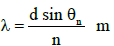(20)

Where, d is the diffraction grating, λ is the wavelength of the incident light, θ is the diffraction angle, and n is the order of the image.

#### Electron’s Circular Magnetic Field (CMF) Diffraction

It is known that, the phase waves or matter waves, exhibit certain striking points of similarity with electromagnetic waves, particularly in their ability to produce the diffraction effects by which they were discovered , and Thomson realized his experiment showed the central spot and rings were deflected together, and they are due to cathode rays of significantly the same velocity , this represents simultaneous deflection characteristic, which is a hint for extraordinary conflicting situation; but not investigated, while G.P. Thomson questioned the nature of these waves? And relation it has with electron associated with it , these scientific concerns were not answered at time; our answer is given by Eq(1), in which any charged in motion produced Circular Magnetic Field (CMF), which is the phase waves, matter waves  or pilot waves ; hence in double slit experiment shown in Figure 3, twenty seven electrons were accelerated by an electron gun, successively towards the two slits; the figure is divided into three sections; first the plan showing the electron gun with the last electron-27 emerging from the gun; the second part is two dimensional perspective of three electrons-26-25-24 surrounded along its trajectory by layers of Circular Magnetic Fields (CMF or BCMF) of varied magnitudes with each moving towards one of the two slits; the third part is a plan shows electron-23 before entering slit-2 it’s CMF is marked with green color, electron-22 is in the middle of slit-1 surrounded by δ-BCMF-1, while part of the CMF- 22 or the δ-BCMF-2 (with lower magnitude ranging between 0.25% to 56.25% of main CMF) showed in middle of slit-2, then electrons-21 just exited from slit-2, while electron-20 before it and both impinged on the monitor, which showed resulted five bright fringes or patches resulted from previous nineteen interferences (19) x (2δ-BCMF-1) of constructive waves that had emerged from both slits.

As electrons emerged from the electron gun, each produced CMF or BCMF along its path, while surrounded by its Electric Field (E-F), shown in two dimension for electrons-26-25 and 24 with magenta color, moving to either slits, the cross sectional plan of electron-23 is shown with CMF and the E-F extended from its center to all sides while approaching slit-2, the CMF (BCMF) intensity is proportional to the velocity as given by Eq(1), and to the acceleration potential as given by Eq(15), thus an electron entered the slit; as shown for electron-22 at the center of slit-1 together with its strongest δ-BCMF-1 this occurred concurrently with other less magnitude portion of its extension δ-BCMF-2 at the center of slit-2, their existence is similar to both δ-Wave entering both slits in Young's double slit experiment shown in Figure 2D, since electron velocity is given in terms of energy by Eq(12), therefore substituting (Ve) from Eq(12) with Ve in Eq(1) then rearranging the equation, the CMF (BCMF) entering slit-1 due to specific energy is given by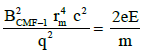(21)

Therefore, from Eq(21), the magnitude of the CMF-1 (BCMF-1) entered a slit with an electron (electron-22 at slit-1 in Figure 3), is given by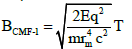(22)

Where, rm is the magnetic radius for (BCMF-1) measured from electron’ center to edge of slit-1, the CMF-2 (BCMF-1) entering a slit without an electron (slit-2 in Figure 3), is given by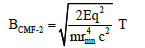(23)

Where, rmn is the magnetic radius for (BCMF-2) measured from electron center to the center of slit-2, it is weaker (by rm- rmm) than BCMF-1 entering slit-1, since a recent double slits experiment suggested that each electron somehow travels through both slits at the same time and interferes with itself, like a wave instead of a particle, resulted in interference pattern , therefore, the existence in Figure 3, of δ-CMF-1 (BCMF-1) in the canter of slit-1 and δ-CMF-2 (BCMF-2) in the center of slit-2, is similar to the existence in Figure2D of δ-Wavelet-1 in the canter of slit-1 and δ-Wavelet-2 in the center of slit-2, and since δ-Wavelet-1 and δ-Wavelet-2 are part of the EM-R been transformed into CMF (BCMF) through the polarization process given by Eq(19), after emerging from both slit-1 and slit-2 respectively, therefore the δ-Wavelet-1 and δ-Wavelet-2 in Figure 3 are the CMF (BCMF), and both CMF represents the same electron producing them, but with slightly different magnitudes, hence both CMF (BCMF) are wrongly perceived.

Figure 3: The diffraction of the Circular Magnetic Field (CMF), produced by an electron. Section 1-shows the last electron 27 emerging from electron gun, 2-two dimensions of three electrons moving towards the two slits surrounded by CMF and EF 3-shows electron-23 before entering the second slit surrounded by CMF, then electronn-22 with δ-BCMF-1 at center of slit-1 and δ-BCMF-2 at slit-2, then electron-21 with CMF impact on the screen together with waves from 1-20. An electro

As both experiments shown in Figures 2 and 3, are characterized in common with δ-BCMF entering both slits, and since both having -BCMF transformed into CMF (BCMF) after emerging from the slit, therefore both CMF (BCMF) formed successive trail of progressive enlarged waves, both caused interference, adding and cancelling each other to form the bright fringe or patches showing in Figure 3, similar to Young's double slit experiment in Figure 2, but this has been perceived and interpreted as waves collapse back into a single particle on screen, which is the foundation problem of quantum mechanics , and as shown there is no magic in this process.

The magnitude of CMF (BCMF) entering any slit in Figures 2 and 3 is given by Eq(9), and for electron-CMF and related CMF entering slit-1 and 2 in Figure 3, it is derived using Eqs (22 and 23) an examples of which is given in Table 3, while the equivalent frequency if transformed into EM-R, for both δ-CMF-1 (BCMF-1) and δ-CMF-2 (BCMF-2) at slit-1 and slit-2 in Figure 3, or both δ-CMF-1 (BCMF-1) and δ-CMF-2 (BCMF-2) in Figure 2, is derived from Eq(9), and given by BCMF-1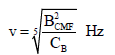(24)

 Slit MagneticRadius CMF(BCMF)10kV(1.60217733e-15J)SEM EquivelantWavelength CMF(BCMF)200kV(3.20435466e-14J)TEM Equivelant Wavelength 1 Slit-1 rm=5.e-6m 1.2669951931923181623917695036668e-9 1.8178473315169439676884838297197e-6 5.6661747582870043765543851017898e-9 9.9850767607941515892337435013263e-7 Slit-2 rmn=1.1e-4m 3.1674879829807954059794237591669e-12 1.9970153521588303178467487002653e-5 1.4165436895717510941385962754475e-11 1.0969211351291313534847121820819e-5 Slit-2/Slit-1% 0.25% 0.25% 2 Slit-1 rm=1.25e-3m 2.0271923091077090598268312058668e-14 1.5062928477142774338129513810871e-4 9.0658796132592070024870161628637e-14 8.2737694458155795363445732167507e-5 Slit-2 rmn=7.5e-3m 5.6310897475214140550745311274079e-16 6.3158297447664341103789267984416e-4 2.5182998925720019451352822674622e-15 3.4691606779197764346705038750261e-4 Slit-2/Slit-1% 2.78% 2.78% 3 Slit-1 rm=3.75e-4m 2.2524358990085656220298124509632e-13 5.7491867728645575234164724489184e-5 1.0073199570288007780541129069849e-12 3.1579148723832170551894633992208e-5 Slit-2 rmn=5e-4m 1.2669951931923181623917695036668e-13 7.2369805764843587033970165095869e-5 5.6661747582870043765543851017898e-13 3.9751306569992391775703472060227e-5 Slit-2/Slit-1% 56.25% 56.25%

Table 3: The relative magnitudes of Circular Magnetic Field One (BCMF-1) and Two (BCMF-2) entering slit-1 and slit-2, respectively in Figure 3, using Eqs. (22) and (23) and acceleration potentials of 10 kV and 200 kV respectively, in (1) rm=5 × 10-6 and rmn=1 × 10-4 , while (2 and 3) are mixture of rmn=5 × 10-3 mm .

The equivalent frequency given by Eq(24), showed that for a CMF wave accompanying an electron to produced intensity similar to EMR, when detected at the monitoring in Figure 3, a minimum intensity is required (can be tested in Table 1), hence this is done by controlling electron velocity or the accelerator potential, the equivalence of BCMF in Eqs. (9 and 22) is given as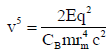(25)

Substituting v withs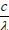in Eq(25), hence the wavelength is given by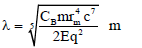(26)

For simplicity Eq(28), is given by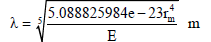(27)

Since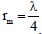substituting this in Eq(26), hence when energy is given, the equivalent wavelength is given by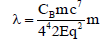(28)

Or for simplicity as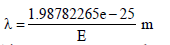(29)

This equation can reproduce the wavelength versus particle energy for electrons given by Kittel , and since the wavelength equal four magnetic radius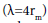substituting this in Eq(28), the Radiation Energy (ER) is given by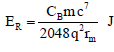(30)

For simplicity, the Radiation Energy (ER) can also be given by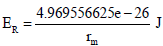(31)

Substituting λ with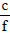in Eq(28), when energy is known, then frequency is given by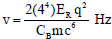(32)

Combined fixed parameters with radiation constant CR, therefore Eq(32) can be written as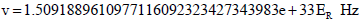(33)

From Eq(33), knowing the frequency v, the energy of any EM-R is given by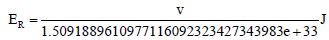(34)

But the inverse of 1.5091889610977116092323427343983e+33 in Eq (34) is the Planck’ formula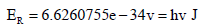(35)

Alternatively, replacing ER in Eq (34) with Planck’ energy formula, the following is obtained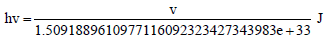(36)

Cancelling the frequency v from both sides of Eq(36), therefore, the given Planck constant , is also given by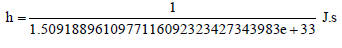(37)

Therefore the inverse of Eq(37), is the Planck’ constant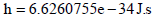(38)

But from Eq(8), the Planck constant is given by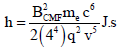(39)

During energy production, the frequency is not the main factor, rather it is the time (tF) , and since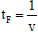,hence Eq (39) becomes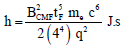(40)

Where, tFis Flipping time in second, but electron mass (m), charge (q) and speed of light (c) in Eq (40) has fixed quantity, the only variables are the CMF (BCMF) and the Flipping Time (tF), and variation of both quantities (BCMF and v) in Eq(40) as given in Table 1, is in a manner to keep the Planck’ constant at fixed magnitude, the products of both the CMF (BCMF) and time (tF) in Eq(40) is given by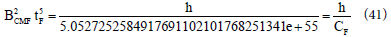Where, 5.0527252584917691102101768251341e+55 is the Fixed constant (CF) From Eq(41), the magnitude of this product is given by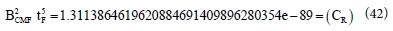But the value 1.3113864619620884691409896280354e −89 is the radiation constant (CR) given in Eq(9), with related data in Table 1, hence Eq(42) shows that, the multiplication of both the CMF (BCMF) and the Flipping time (tF) produced constant value for any EM-R wave, therefore from Eqs. (41) and (42) the Plank’ constant represents the following two constants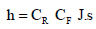(43)

Therefore, the multiplication of the Fixed constant (CF) in Eq (41) by radiation constant (CR) given by multiplication of both CMF (BCMF) and the Flipping time (tF), for any of their value in Table 1, produced the Planck’ constant h, therefore Planck’ constant existed in the combined value of both the CMF (BCMF) and the Flipping time (tF), it only emerged when Fixed constant (CF) is multiplied by radiation constant (CR) as given in Eq(43), therefore, the origin of Planck’ constant can be expressed as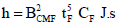(44)

Therefore, the Planck’ constant can also be given by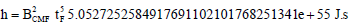(45)

Multiplying both part of Eq(45) by frequency v, the Radiation Energy (ER) is given by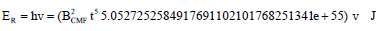(46)

Changing the frequency (v) in Eq(46) with time (tF), therefore the Radiation Energy (ER) is given by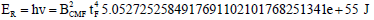(47)

This radiation energy can be expressed bys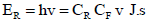(48)

#### Results and Discussion

Suggestions are made regarding relation between Electromagnetic Radiation (EM-R) entering and emerging from small hole or slits:

- When entering and emerging from slit/hole, the EM-R lost the electric field due to polarization effect.

- The wavelet which entered and emerged from slits is the magnetic part of the EM-R.

- When emerged from the hole or slit this magnetic wave becomes circular in shape.

- The resulted Circular Magnetic Field (CMF), lost characteristics of EM-R radiation, particularly the speed of light, as electric field is lost.

The shape of a diffracted wave on screen due to a single slit/hole is representation of the emerged CMF, while waves from two slits interfere constructively or destructively.

The double-slit experiment, or the electron diffraction, it is described as the entry of CMF from one electron into two slits, with varied magnitudes, of CMF accompanied by the electron through a slit having greater magnitude.

The ceased of interference pattern when detector is put near one of the slits to determine which slit(s) an electron is passing through, at which electrons create two straight lines, like classical particles , as shown in Figure 3, such detection interfere with the electrons CMF and passing through both slits gives the lines.

As showed by Eq(1), the CMF produced by such electron is the main energy of electromagnetic radiation wave, therefore the so-called phase waves or matter waves or plot wave which exhibit certain striking points of similarity with electromagnetic waves, particularly in their ability to produce the diffraction effects by which they were discovered , is just a CMF.

It is clear G.P. Thomson was correct right from the beginning in realizing de Broglie’s theory as a theory of light and electronic orbits, not as a theory of electron diffraction .

Planck’ constant is related to the double slit experiment by the energetic CMF and relation with energy production.

As shown in Eq(43) Planck’ constant consists of two constants, the fixed (m, q and c) parameters of the energy formula designated as Fixed Constant (CF) and the two variables the CMF (BCMF) and the Flipping time (tF) designated as Radiation Constant (CR).

The multiplication of Eq.(47) by t4 gives the radiation energy, while multiplication of the same equation be t5 gives the Planck’ constant, as in Eq(44).

Therefore, Planck’ constant is the variation of Radiation Constant (CR), which contains both the CMF (BCMF) and the Flipping time (), the multiplication of which gives a constant value for each radiation, and it’s multiplication by the Fixed Constant parameters (CF) produced the Planck’ constant (h).

It is clear from Eq(44), that Planck in expressing that his constant is merely a mathematical trick to obtain the right description (formula) of the black body radiation spectral intensity profile 

#### Conclusion

The disclosed knowledge of the Magnetic Force (Fm) , Spinning Magnetic Field (SMF), and the produced Spinning Magnetic Force (SMFc) , allowed the Flip-Flop (F-F) mechanism for Electromagnetic Radiation (EM-R) , and reproduced the Radiation Magnetic Force (FmR) formula showed an embedded force in EM-R similar in nature to Planck’ energy, thus excluding quanta (photon) in removing electron from atom in Photoelectric Effect , the F-F condition and parameters for the speed of light was derived , showed Compton Effect as an internal production of Secondary EM-R (S-EM-R), with the existence of Electromagnetic Radiation Force (FEMR), pulling the produced EM-Wave (EM-W) at the ends of F-F mechanism . These background lead to the suggestion that, the diffraction phenomenon is due to a change in characteristics of EM-R, resulted in a wave neither travel with speed of light, nor carrying electric field, the wave is not semi-circle, rather it is a full circular wave, and composed of the magnetic part of the EM-R, or the Circular Magnetic Field (CMF), originated from accelerated electrons  given by Eq (1), therefore the passage of light through single hole/slit resulted in rings on the monitor, while the interference of two such CMF in double slits experiment, produced constructive or destructive interference, shown as patches on the screen. Thus electron diffraction in double slit experiment is interpreted as the entry and emerged of CMF in and out of both slits/ holes in addition to the electron producing them, thus both CMF produced constructive or destructive interference; the origin of the Planck’ constant (h) is finally derived, and showed to form relationship between two constants, the Radiation constant (CR), composed of both the CMF (BCMF) and the Flipping time (tF), and the Fixed constant (CF), the Planck’ constant resulted from the multiplication of both constants.

Finally, G.P. Thomson found it impossible to explain his results “except by the assumption of some kind of diffraction” [11,23], simply because he faced what Compton faced before , contrary to Compton, Davisson and Germer and Thomson, who followed Einstein quanta (photon) line [5,9,11], we realized Raman understood the problem by early stating that “the classical wave-principles are not easily reconcilable with Compton effect because they have not been correctly interpreted,” ; the simplistic explanation of billiard-ball of quanta, allowed the emergence of such complicated ideas and alleged predication by Quantum Mechanics (QM) that any detector capable of determining the path taken by a particle through one or the other of a two-slit plate will destroy the interference pattern , such line of thoughts empowered some to think QM represents the super knowledge, even an attempt has been made to establish relation between it and higher brain functions , leading some to imagine QM as a steppingstone between ourselves and the Universe, between what we want and making it actually happen in the natural ; hence what QM succeeded to attained was to get rid of common sense because as it claimed common sense makes a lot of mistaken assumptions , but as seen two great historical lessons could be draw from this experience that, the collection of lots of data without being able to find any basic underlying principles is not science , and science is an open field any individual can explore for the benefit and progression of humankind.

#### References

Select your language of interest to view the total content in your interested language

### Article Usage

• Total views: 12493
• [From(publication date):
June-2016 - Nov 14, 2019]
• Breakdown by view type
• HTML page views : 12165Can't read the image? click here to refresh